### Why should I choose AnalystNotes?

Simply put: AnalystNotes offers the best value and the best product available to help you pass your exams.

##### Subject 5. The Future Value and Present Value of a Single Cash Flow
When you make a single investment today, its future value, received N years from now, is as follows:• FV = future value at time n
• PV = present value
• r = interest rate per period
• N = number of years

A key assumption of the future value formula is that interim interest earned is reinvested at the given interest rate (r). This is known as compounding.

In order to receive a single future cash flow N years from now, you must make an investment today in the following amount: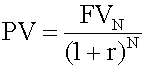Notice that the future cash flow is discounted back to the present. Therefore, the interest rate is called the discount rate.

You should be able to calculate PVs and FVs using your calculator.

• N = number of periods
• I/Year = yield in market place or the required rate of return
• PV = present value
• PMT = payment amount per period
• FV = the future value of the investment

One can solve for any of the above variables. Just input the other variables and solve for the unknown. Using the calculator on the test will prove to be a very time-efficient manner of calculating present values and future values.

Example 1

An analyst invests \$5 million in a 5-year certificate of deposit (CD) at a local financial institution. The CD promises to pay 7% per year compounded annually. The institution also allows him to reinvest the interest at the same CD rate for the duration of the CD. How much will the analyst have at the end of five years if his money remains invested at 7% for five years with no withdrawals of interest?

Before using the Texas Instruments BAII PLUS and HP 12C calculator, it is essential to ensure that your settings are correct. The default settings on the calculator are not necessarily the settings you need when making the calculations. Follow these steps to ensure that your calculator is correctly set.

Texas Instruments BAII PLUS Settings

• Press 2nd QUIT 2nd [CLR TVM] to clear the worksheet.
• Press 2nd [P/Y] to enter payments per year and/or compounding periods per year.
• The P/Y label and current value are displayed. The default value is 12. You must now key in 1 and then ENTER since you want 1 payment per year.
• If the question says there are 12 payments per year, you would change this to 12.

HP 12C Settings

• Turn the calculator on by pressing the ON key.
• Clear the memory and set decimals to 2 places by pressing the following keys:
• CLEAR REG f 2 - 0.00 will display.
• CLEAR FIN - this clears all the data in the financial mode.

The calculator keys to press are: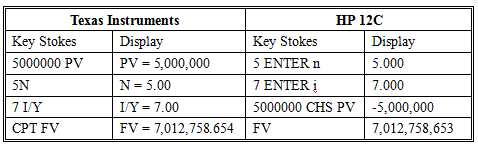If you are given the FV and need to solve for PV, the calculator keys to press are:When compounding periods are not annual

Some investments pay interest more than once a year. When you calculate these amounts, make sure that periodic interest rates correspond to the number of compounding periods in the year. For example, if time periods are quoted in quarters, quarterly interest rates should be used.

When compounding periods are other than annual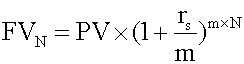• rs = the quoted annual interest rate
• m = the number of compounding periods per year
• N = the number of years.

Example 2

An analyst invests \$5 million in a 5-year certificate of deposit (CD) at a local financial institution. The CD promises to pay 7% per year, compounded semi-annually. The institution also allows him to reinvest the interest at the same CD rate for the duration of the CD. How much will the analyst have at the end of five years if his money remains invested at 7% for five years with no withdrawals of interest?

The calculator keys to press are: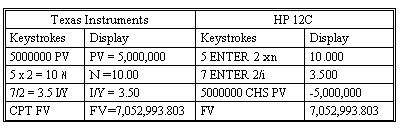Note that the answer is greater than when the compounding was annual. This is because interest is earned twice a year instead of only once.

If the number of compounding periods becomes infinite, interest is compounding continuously. Accordingly, the future value N years from now is computed as follows: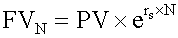User Comment
Nathan I would add here that adjusting the number of periods per year, 2nd [P/Y], gets cumbersome. Use the given periodic interest rate (other) and enter Years/m for N. That procedure tends to be faster and doesn't involve hidden settings that could throw off all of your calculations if you forget to make the adjustment each time.
dg18sox when I enter the semi-annual compounding into my HP 12c using PV -\$5mm, i = .07/2 = .035, n= 5 * 2= 10. I receive a FV = \$5,017,527.588 which I know to be incorrect, because I get the same answer as when I do the formula out.

Does anyone know what I'm doing wrong on the calculator?
spattewar keep the [P/Y] setting to 1. and then I/Y = 7/2 = 3.5, N = 5*2 =10, PV = 5,000,000

CPT FV
Taimoor Continuous compounding using TI BAII Plus:
0.07 * 5 [2ND] --> [LN (ex)] = 1.4191 * 5,000,000
gives
7,095,337.74
quantwannabe I don't know what to say but I have found few numbers here are wrong. what I got when I crunched a number on my H12c Platinum calculator are as following:

1. Compounding Annually

5 million was invested at the 7% annual rate for 5 year CD.

step 1. enter f and FIN (to clear financial register data)
Step 2. enter 5,000,000, CHS and PV
Step 3. enter 7 and i
Step 4. enter 5 and n
Step 5. enter FV that would give you 7,012,758.654

2. Compounding other than annual (This one is right on this web)

5 million was invested at the 7% annual rate compounded semiannually for 5 year CD.

Step 1. enter f and FIN (to clear financial data memory)
Step 2. enter 5,000,000, CHS, and PV
Step 3. enter 3.5 (because for six month period the rate is half of 7% annual rate)
Step 4. enter 10 (there are 10 six months period in five years)
Step 5. enter FV that would give you 7,052,993.803
netty For compunding annually i used my own calculator using the formula method and got a different answer whereas for compounding other than annual i used my calculator n the formula method and arrived at the same answer u got why?
tilleryj Following the instructions for the TI, I always end up with negative values for FV and PV. Does anyone know what might be causing this?
madhi The present value should be entered into the calculator as a negative number because it is considered as cash outflow you're making, then, you should get your positive future value at the end of the investment life (i.e. Cash inflow).
babyheaven234 In the formula for compounding continuously the "e" stands for exponential in logrithms.
Shalva It doesn't matter whether PV is negative or FV. The inherent idea behind each TVM problem, is that PV and FV have different signs. One of them is cash inflow, and other - cash outflow
peteypete you don't need a financial calculator to do this...
oncewerepirates But you have a use a financial calculator in the exam... And these financial calculators are nowhere near as clear to use as my old Casio calculators...
Andystar @madhi: I don't think that's right, compounding will just give you the compounded outflow, you can take FV-PV to find the accrued interest instead
chipster When I do this on my TI BA II Plus I get the correct number but a negative sign--can't figure out why...anyone know? Thanks
sammyGyan am really happy and to hear of all this. guys am yet to start this whole process.hope any of you could help me out.thanks
bmalbra Why do i need to keep the setting to 1 P/YR when i am doing the semi-annual calculation. Shouldn't it be 2 p/yr. Based on the answer given, that would be incorrect, but why?

I thought we change the settings as follow
annually 1 P/YR
Semi-annual 2 P/yr
Quarterly 4 p/yr
monthly 12 p/yr

Thank you so much